# Region Proposal Network (RPN) : A Complete Guide

This tutorial includes detailed step by step guide of how Region Proposal Network (RPN) works. It is mainly used in RCNN family for object detection. Those who are familiar with RCNN, they might already have encountered the term `Region Proposal Network`. Before we get into details of RPN, let's understand how object detection works using R-CNN.
Object Detection using R-CNN

R-CNN is a Region based Convolutional Neural Network. It is a state of art architecture for object detection. Let's say you have a photograph, the goal of objective detection is to detect cars and people in the photograph. There are a couple of cars and people in the photograph so we need to detect all of them.

How does it work?
1. Region Proposal

Extract many regions from an image using Selective Search. 2000 regions were used in the original whitepaper. Regions are drawing multiple bounding boxes in the input image. See the yellow bordered boxes in the image below.

2. Calculate CNN Features

Create feature vector for each region proposed using CNN network trained for classifying image

3. Classify Regions

Classify each region using linear Support Vector Machine model for each category by passing feature vector generated in the previous step.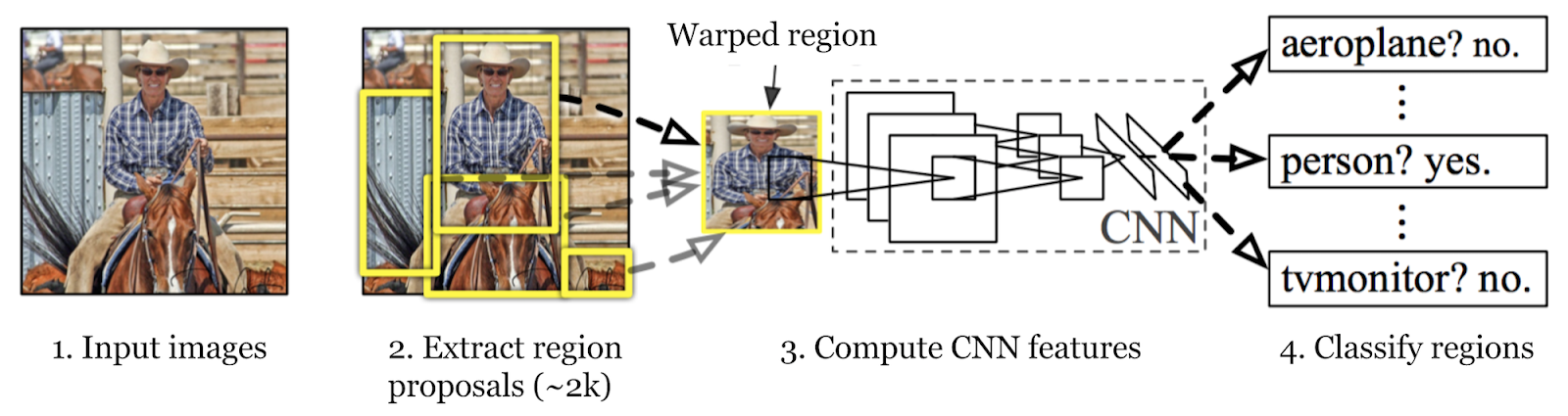## Region Proposal Network : What does it do?

In R-CNN we have learnt extracting many regions using Selective Search. The problem with the Selective Search is that it's an offline algorithm and computationally expensive. Here Region proposal network comes into picture. In Faster R-CNN, Region Proposal Network were introduced to use a small network to generate region proposals. RPN has classifier that returns the probability of the region. It also has regressor that returns the coordinates of the bounding boxes.

Following are the steps involved in Region Proposal Network

1. Convolutional Neural Network

Let's say you have an image shown below.

The image is represented as `Height x Width x Depth` multidimensional arrays. Notation of width as w, height as h, depth as (x,y)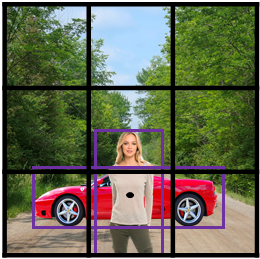The input image is passed through Convolutional Neural Network. Final layer of the CNN returns the convolutional feature map.

2. Generate anchor boxes

Anchor box is a box surrounding the objects (or called bounding box). We manually define the box and label its object class. This box has a certain height and width which is selected based on the heights and widths of objects in a training dataset.

Let's take two anchor boxes for demonstration purpose. Shape of Anchor Box 1 and box surrounding car is very much alike. Similarly shape of Anchor Box 2 and box around girl are similar.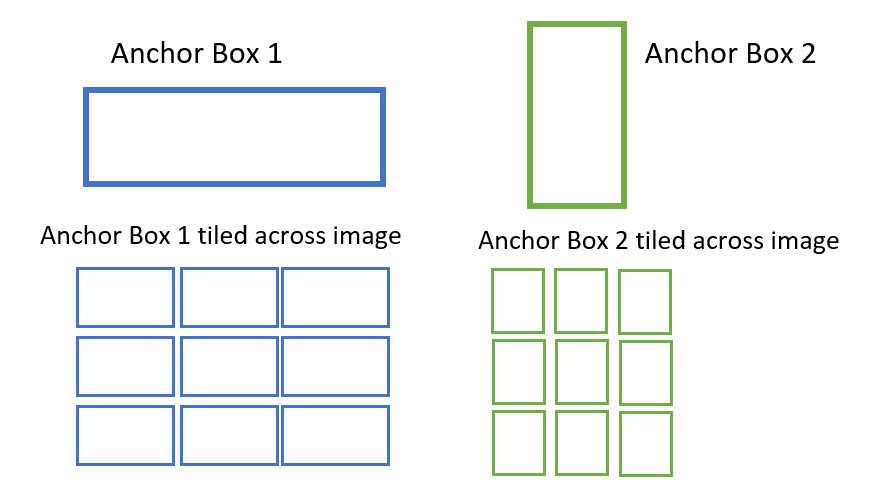Remember we are making use of convolutional feature map as explained in the previous step. We will position anchor boxes for each position of the convolutional feature map to the input image. It returns tiled anchor boxes across the entire image (as shown above). Each anchor box produces prediction if it has our desired object or not. Prediction is in the form of binary classification. Let's say if the anchor box contains desired object it would be classified as "foreground" and rest would be background. Since we have two anchor boxes, we will have two predictions per position. Please make a note that some of the anchor boxes won't have our desired object as there are so many boxes.

3. Classify anchor box as foreground/background

To classify each anchor box, we use Intersection over Union (IOU) distance metric. It is used to describe the extent of overlap of anchor box with the desired object. The greater the region of overlap, the greater the IOU. If anchor box having IOU greater than 0.5, it will be considered foreground and those having less than 0.1 IOU are considered background.

What is "desired" object?

It is the target object which you want as output from the deep learning model. In deep learning it is called ground truth object.

In this step regression layer tries to make the coordinates of the bounding box around the desired object more precise. Coordinates of the object are width, height and depth (or called center).

Loss function of Regional Proposal Network is the sum of classification (cls) and regression (reg) loss. The classification loss is the entropy loss on whether it's a foreground or background. The regression loss is the difference between the regression of foreground box and that of ground truth box.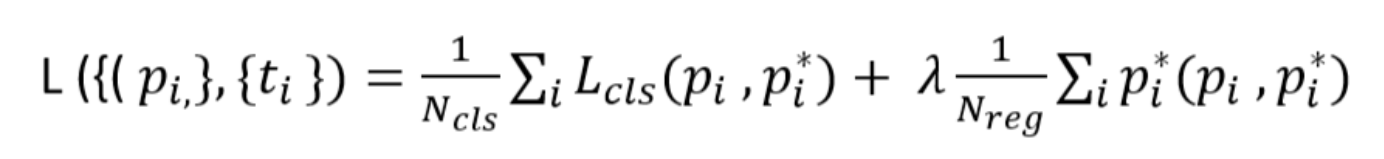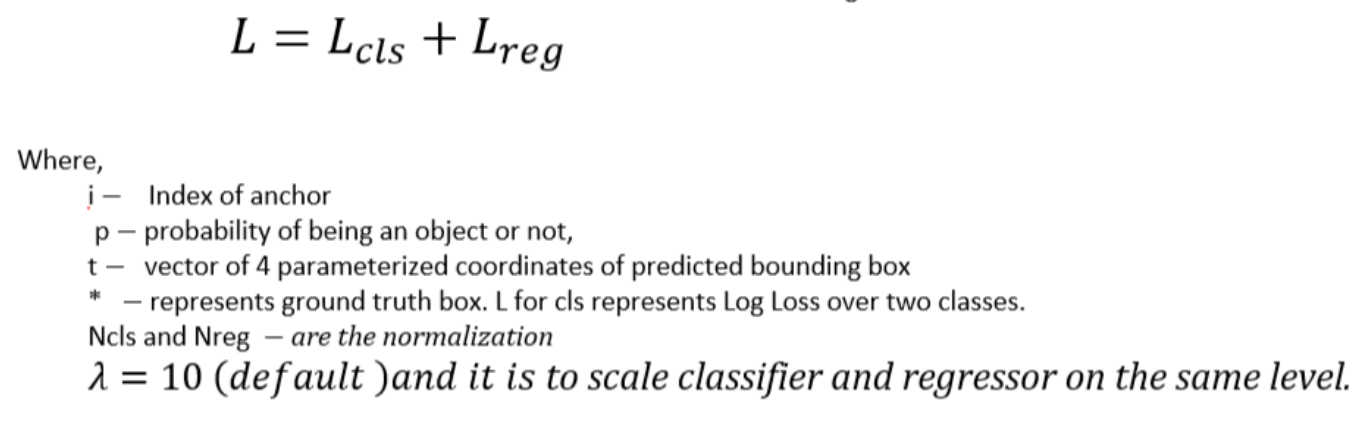RPN does not return whether the object is car or girl. It only tells whether it is foreground or background.
Important Points
How to determine Anchor Box Size?

In general we take anchor boxes of size - 64x64, 128x128 and 256x256. One should consider sizes that closely represent the scale and aspect ratio of objects in your training data. Statistically one can calculate K-means to group similar anchor boxes.

How to choose the number of Anchor Boxes?

Calculate k-means clustering with the IOU distance metric (instead of Euclidean distance). Then compute mean IOU of the anchor boxes in each cluster. Loop through the number of anchor boxes and store the mean IOU against the number of anchor boxes. Then decides the number of anchor boxes based on the incremental mean IOU by adding more boxes.

Summary

RPN was proposed to solve the limitations of Selective Search which are offline algorithm and computationally expensive. RPN is more efficient.
If RPN needs to be summarised briefly it will be "Image passes through CNN and get feature map. For each position in the feature map, you have anchor boxes and every anchor box has two possible outcomes - foreground and background."

Related Posts
Share